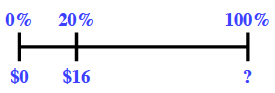### Home > MC2 > Chapter 7 > Lesson 7.2.2 > Problem7-81

7-81.

Alex has a job delivering newspapers. He puts $20\%$ of his earnings each week into his college savings account. Each week he puts $16$ into the account.

1. Draw a diagram to represent this situation. How much money does Alex earn each week?$16$ is $20\%$ of what?

$\frac{20}{100}=\frac{16}{\textit{x}}$

$80$

2. Alex spends $10\%$ of his earnings on snacks each week. How much does he spend?

Find $10\%$ of the answer from part (a).

$0.10$ $\left(80\right)$

$8$

3. When Alex has worked for one year, he will get a raise that is equal to $15\%$ of his current earnings. How much more money will he earn each week?

Find $15\%$ of earnings per week, the answer from part (a).